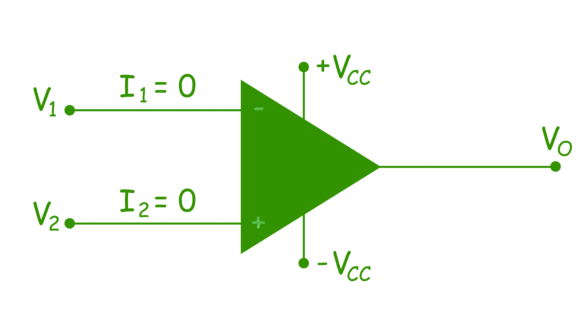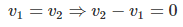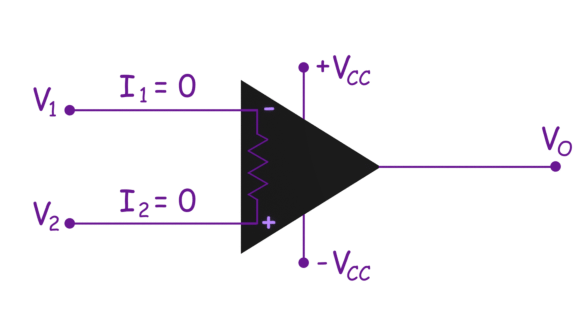# Ideal Operational Amplifier or Ideal O Amp

## What is an Ideal OP Amp?

An operational amplifier (OP Amp) is a direct current coupled voltage amplifier. That is, it increases the input voltage that passes through it. The input resistance of an OP amp should be high whereas the output resistance should be low. An OP amp should also have very high open loop gain. In an ideal OP amp, the input resistance and open loop gain is infinity whereas the output resistance is zero.

An ideal OP amp has have following characteristics—

 Characteristic Value Open Loop Gain (A) ∝ Input Resistance ∝ Output Resistance 0 Bandwith of Operation ∝ Offset Voltage 0

So, an ideal op amp is defined as, a differential amplifier with infinite open loop gain, infinite input resistance and zero output resistance.

The ideal op amp has zero input current. This is because of infinite input resistance. As the input resistance of ideal op amp is infinite, an open circuit exists at input, hence current at both input terminals is zero.There is no current through the input resistance, there will be no voltage drop between the input terminals. Hence no offset voltage appears across the inputs of an ideal operational amplifier.

If v1 and v2 are the voltages of inverting and non-inverting terminals of op amp, and v1 = v2 then in ideal case,The bandwidth of operation of an ideal op-amp is also infinite. That means the op-amp perform its function for all ranges of frequencies of operation.

Want To Learn Faster? 🎓
Get electrical articles delivered to your inbox every week.
No credit card required—it’s 100% free.